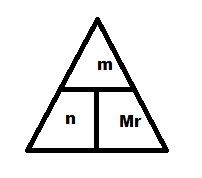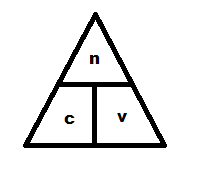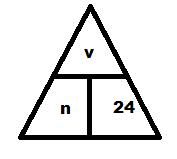How do I work out the Mole of a substance?

I'm doing a GCSE Chemistry unit two exam in January and I'm really bad at remembering how to work out things such as moles, empirical formula etc... so if anyone has any ideas of how I could remember them it would be much appreciated :)

So my question is 'How do I work out the Mole of a substance?"

Bear in mind that chemistry is my major weakness, so step by step with clear instructions would be best please...

thanks!

Posted Sat 29th December, 2012 @ 12:22 by Stargirl
Edited by Stargirl on Sat 29th December, 2012 @ 12:23

Use these triangles :)n=no moles, m=mass (g), Mr=relative molecular mass (also works for atomic mass), c=concentration, v=volume (dm^3)

Top one is for solids, middle for solutions and bottom for gases (although you probably won't need that one at GCSE)

Answered Wed 2nd January, 2013 @ 13:27 by Lucy Carr
Edited by Lucy Carr on Wed 2nd January, 2013 @ 13:28

moles = mass divided by mr (molecular mass)

Answered Sat 29th December, 2012 @ 13:12 by Sohail

The formula of moles=mass/Mr is used for calculating the moles of a solid. In order to calculate the number of moles in solution, you need to use the formula: moles=concentrationxvolume (where concentration is in moldm^-3 and volume is in dm^-3.

Both of these can be rearranged, and if you like they can be place into formula triangles to help you with that.

You then need to find what you know, so the mass, volume or concentration will probably be told to you, and you may have to work out Mr using your periodic table and the formula of the substance. You then just put everything into the formula, and hopefully, you will get the answer you are after!

Answered Sat 29th December, 2012 @ 18:09 by Hannah

Moles in a Solid = Mass / Relative Formula Mass

Moles in a Solution = (Volume (cm3) x Concentration) / 1000

Moles in a Gas = Volume (dm3) / 24 OR Volume (cm3) / 24000

Answered Sat 29th December, 2012 @ 20:29 by KatieL